Ben Orlin is the author of Math with Bad Drawings and Change is the only Constant: The Wisdom of Calculus in a Madcap World.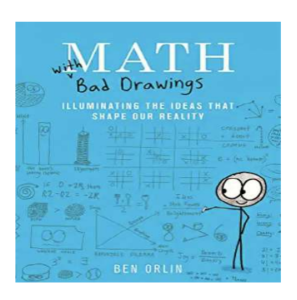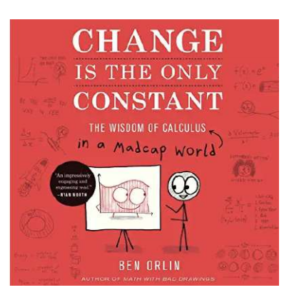Besides have a good sense of humor, Ben is good at recognizing the differences in the way students and mathematicians see math problems.

Take for example, the math problem, What is 7 x 11 x 13.

What goes through your mind when you see a problem like that?

I reach for my calculator, or might try 7 x 11 first, then look for a pen a paper, but what Ben suggests is that mathematicians imagine this.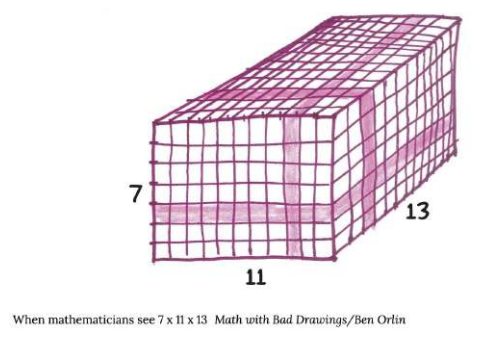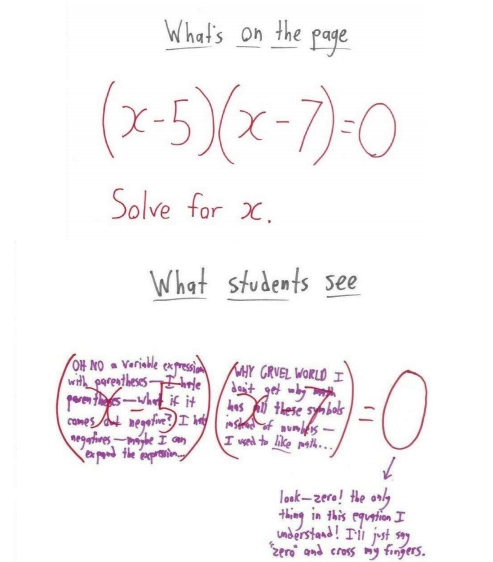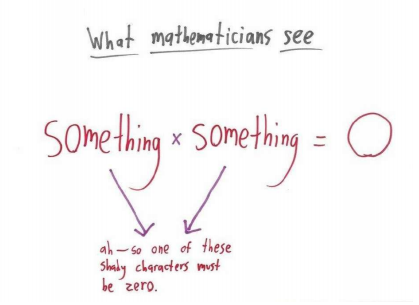The mathematician’s simplification involves recognizing a pattern. But how often are students explicitly taught to look for patterns that can help students sort the types of problems they are seeing? Some teachers and textbooks may be doing so, but many do not.

Many dyslexic students may learn math best through inductive reasoning, observe examples of correctly solved problems, then reasoning backwards to general principles. If they miss out on understanding the different types of problems, then they can be utterly lost, struggling to match different problem solving steps to the right problems.

If you are tutoring a student, verbally labeling the pattern (maybe even color coding) may help students recognize patterns.

The above example may not happen so often, but when our kids were studying for the PSAT, labeling the patterns of questions they were likely to see helped a lot. If it’s not clear what “type” of question you’re seeing, looking at the titles of chapter subheadings in a syllabus or textbook may help.

Below is another good example of how students can be lost in details, whereas a mathematician can grasp the big picture that is being asked so that a problem can be solved by reasoning without going through number and symbol manipulations.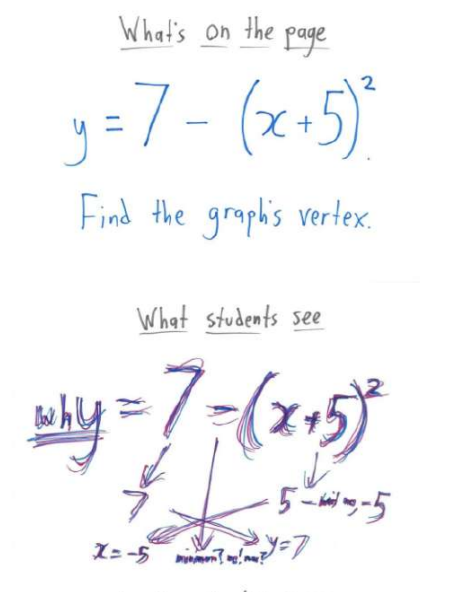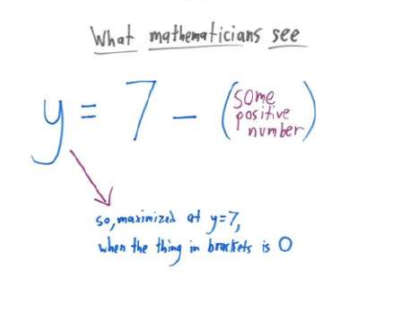I wish I could say that Ben’s Bad Drawings book could be a detailed guide for tutors or teachers working with struggling students, but unfortunately it has too few examples for those who may need more substantial guidance.

Math teachers may find it helpful though and students who are struggling may find it encouraging to read his cartoons.

Check out another excerpt from Ben’s book to see how mathematicians might see the formula for an area of a circle. Because many dyslexic students need to understand the basic reasoning why certain formulas work, one way of explaining why for a circle, A = πr2 is to follow Ben’s reasoning as presented in the Popular Science article HERE.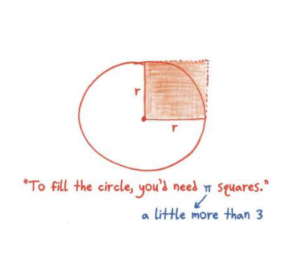Clearly, the circle is bigger. But it’s not quite four times bigger (since four squares would cover the circle and then some). Eyeballing it, you might speculate that the circle is a little more than three times bigger than the square.

And that’s exactly what our formula says:
Area = a little more than 3 × r².

If you want to verify the precise value—why 3.14-ish and not 3.19-ish?—then you can use a “proof.”